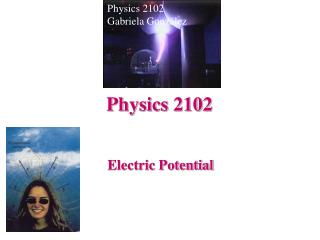DownloadDownload PresentationPhysics 2102

# Physics 2102

Download Presentation## Physics 2102

- - - - - - - - - - - - - - - - - - - - - - - - - - - E N D - - - - - - - - - - - - - - - - - - - - - - - - - - -
##### Presentation Transcript

1. Physics 2102 Gabriela González Physics 2102 Electric Potential

2. Electric potential energy, electric potential Electric potential energy of a system = = - work (against electrostatic forces) needed to needed to build the system U= - W Electric potential difference between two points = work per unit charge needed to move a charge between the two points:DV = Vf-Vi = -W/q

3. Electric potential energy, electric potential Units : [U] = [W]=Joules; [V]=[W/q] = Joules/C= Nm/C= Volts [E]= N/C = Vm 1eV = work needed to move an electron through a potential difference of 1V: W=qDV = e x 1V = 1.60 10-19 C x 1J/C = 1.60 10-19 J

4. Electric field lines and equipotential surfaces • Given a charged system, we can: • calculate the electric field everywhere in space • calculate the potential difference between every point and a point where V=0 • draw electric field lines • draw equipotential surfaces

5. -Q +V +Q 0 -V Equipotential Surfaces & Electric Field • In a uniform electric field E, equipotentials are PLANES. • Electric field points towards lower potential. • In a gravitational field, a free mass moves from high to low potential. In an electric field, which of the following is true? (a) Positive charge moves to lower V, negative charge moves to higher V (b) Positive charge moves to higher V, negative charge moves to lower V (c) All charge moves to lower V. Note: all charges freely move to regions of lower potential ENERGY! Don’t confuse potential with potential energy!

6. Electric Potential of a Point Charge Potential = V = “Work you have to do to bring +1 C from infinity to distance r away from a point charge Q” Note: if Q were a negative charge, V would be negative r

7. q4 q3 r3 r4 r2 r5 q2 q5 r1 P q1 Electric Potential of Many Point Charges • Electric potential is a SCALAR • Just calculate the potential due to each individual point charge, and add together! (Make sure you get the SIGNS correct!)

8. a +Q -Q r Electric Potential of a Dipole (on axis) What is V at a point at an axial distance r away from the midpoint of a dipole (on side of positive charge)? Far away, when r >> a: V

9. Electric Potential on Perpendicular Bisector of Dipole You bring a charge of -3C from infinity to a point P on the perpendicular bisector of a dipole as shown. Is the work that you do: • Positive? • Negative? • Zero? a +Q -Q P -3C

10. -Q +Q Electric Potential of Many Point Charges What is the electric potential at the center of each circle? • Potential is a SCALAR • All charges are equidistant from each center, hence contribution from each charge has same magnitude: V • +Q has positive contribution • -Q has negative contribution A: -2V+3V = +V B: -5V+2V = -3V C: -2V+2V = 0 A B C Note that the electric field at the center is a vector, and is NOT zero for C!

11. R Continuous Charge Distributions • Divide the charge distribution into differential elements • Write down an expression for potential from a typical element -- treat as point charge • Integrate! • Simple example: circular rod of radius R, total charge Q; find V at center. dq

12. x dx a L Potential of Continuous Charge Distribution: Example • Uniformly charged rod • Total charge q • Length L • What is V at position P shown? P

13. Summary: • Electric potential: work needed to bring +1C from infinity; units = V • Electric potential uniquely defined for every point in space -- independent of path! • Electric potential is a scalar -- add contributions from individual point charges • We calculated the electric potential produced: • by a single charge: V=kq/r, • by several charges using superposition, and • by a continuous distribution using integrals.